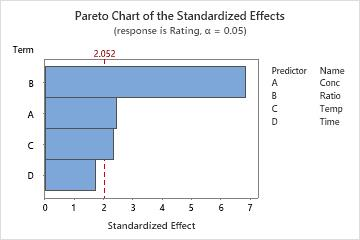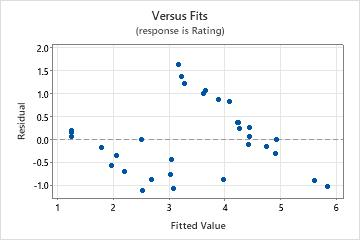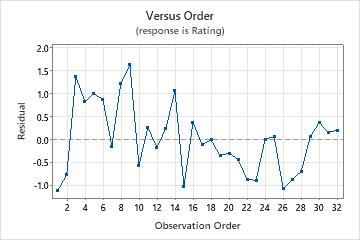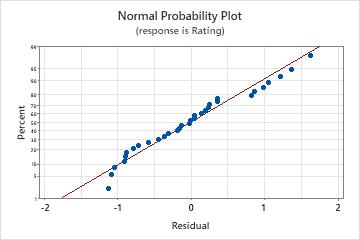# Interpret the key results for Fit Regression Model

Complete the following steps to interpret a regression model. Key output includes the p-value, the coefficients, R2, and the residual plots.

## Step 1: Determine which terms contribute the most to the variability in the response

Use a Pareto chart of the effects to compare the relative magnitude and the statistical significance of the terms. The chart appears when the model leaves degrees of freedom for error.

Minitab plots the terms in decreasing order of their absolute values. The reference line on the chart indicates which terms are significant. By default, Minitab uses a significance level of 0.05 to draw the reference line.Key Results: Pareto ChartIn these results, the effects for 3 terms are statistically significant (α = 0.05). The significant effects are formaldehyde concentration (A), catalyst ratio (B), and temperature (C). The effect for time (D) is not statistically significant because the bar does not extend past the red line. The largest effect is catalyst ratio (B) because the bar extends the farthest. the effect for time (D) is the smallest because the bar extends the least.

## Step 2: Determine whether the association between the response and the term is statistically significant

To determine whether the association between the response and each term in the model is statistically significant, compare the p-value for the term to your significance level to assess the null hypothesis. The null hypothesis is that there is no association between the term and the response. Usually, a significance level (denoted as α or alpha) of 0.05 works well. A significance level of 0.05 indicates a 5% risk of concluding that an association exists when there is no actual association.
P-value ≤ α: The association is statistically significant
If the p-value is less than or equal to the significance level, you can conclude that there is a statistically significant association between the response variable and the term.
P-value > α: The association is not statistically significant
If the p-value is greater than the significance level, you cannot conclude that there is a statistically significant association between the response variable and the term. You may want to refit the model without the term.
If there are multiple predictors without a statistically significant association with the response, you can reduce the model by removing terms one at a time. For more information on removing terms from the model, go to Model reduction.
If a model term is statistically significant, the interpretation depends on the type of term. The interpretations are as follows:
• If a continuous predictor is significant, you can conclude that the coefficient for the predictor does not equal zero.
• If a categorical predictor is significant, you can conclude that not all the level means are equal.
• If an interaction term is significant, you can conclude that the relationship between a predictor and the response depends on the other predictors in the term.
• If a polynomial term is significant, you can conclude that the data contain curvature.

## Step 3: Determine how well the model fits your data

To determine how well the model fits your data, examine the goodness-of-fit statistics in the Model Summary table.

S

Use S to assess how well the model describes the response. Use S instead of the R2 statistics to compare the fit of models that have no constant.

S is measured in the units of the response variable and represents how far the data values fall from the fitted values. The lower the value of S, the better the model describes the response. However, a low S value by itself does not indicate that the model meets the model assumptions. You should check the residual plots to verify the assumptions.

R-sq

The higher the R2 value, the better the model fits your data. R2 is always between 0% and 100%.

R2 always increases when you add additional predictors to a model. For example, the best five-predictor model will always have an R2 that is at least as high as the best four-predictor model. Therefore, R2 is most useful when you compare models of the same size.

Use adjusted R2 when you want to compare models that have different numbers of predictors. R2 always increases when you add a predictor to the model, even when there is no real improvement to the model. The adjusted R2 value incorporates the number of predictors in the model to help you choose the correct model.

R-sq (pred)

Use predicted R2 to determine how well your model predicts the response for new observations. Models that have larger predicted R2 values have better predictive ability.

A predicted R2 that is substantially less than R2 may indicate that the model is over-fit. An over-fit model occurs when you add terms for effects that are not important in the population. The model becomes tailored to the sample data and, therefore, may not be useful for making predictions about the population.

Predicted R2 can also be more useful than adjusted R2 for comparing models because it is calculated with observations that are not included in the model calculation.

AICc and BIC
When you show the details for each step of a stepwise method or when you show the expanded results of the analysis, Minitab shows two more statistics. These statistics are the corrected Akaike’s Information Criterion (AICc) and the Bayesian Information Criterion (BIC). Use these statistics to compare different models. For each statistic, smaller values are desirable.
Consider the following points when you interpret the goodness-of-fit statistics:
• Small samples do not provide a precise estimate of the strength of the relationship between the response and predictors. For example, if you need R2 to be more precise, you should use a larger sample (typically, 40 or more).

• Goodness-of-fit statistics are just one measure of how well the model fits the data. Even when a model has a desirable value, you should check the residual plots to verify that the model meets the model assumptions.

## Step 4: Determine whether your model meets the assumptions of the analysis

Use the residual plots to help you determine whether the model is adequate and meets the assumptions of the analysis. If the assumptions are not met, the model may not fit the data well and you should use caution when you interpret the results.

For more information on how to handle patterns in the residual plots, go to Residual plots for Fit Regression Model and click the name of the residual plot in the list at the top of the page.

### Residuals versus fits plot

Use the residuals versus fits plot to verify the assumption that the residuals are randomly distributed and have constant variance. Ideally, the points should fall randomly on both sides of 0, with no recognizable patterns in the points.

The patterns in the following table may indicate that the model does not meet the model assumptions.
Pattern What the pattern may indicate
Fanning or uneven spreading of residuals across fitted values Nonconstant variance
Curvilinear A missing higher-order term
A point that is far away from zero An outlier
A point that is far away from the other points in the x-direction An influential point
In this residuals versus fits plot, the points do not appear to be randomly distributed about zero. There appear to be clusters of points that could represent different groups in the data. You should investigate the groups to determine their cause.### Residuals versus order plot

Use the residuals versus order plot to verify the assumption that the residuals are independent from one another. Independent residuals show no trends or patterns when displayed in time order. Patterns in the points may indicate that residuals near each other may be correlated, and thus, not independent. Ideally, the residuals on the plot should fall randomly around the center line:If you see a pattern, investigate the cause. The following types of patterns may indicate that the residuals are dependent.
In this residuals versus order plot, the residuals do not appear to be randomly distributed about zero. The residuals appear to systematically decrease as the observation order increases. You should investigate the trend to determine the cause.### Normal probability plot of the residuals

Use the normal probability plot of the residuals to verify the assumption that the residuals are normally distributed. The normal probability plot of the residuals should approximately follow a straight line.

The patterns in the following table may indicate that the model does not meet the model assumptions.
Pattern What the pattern may indicate
Not a straight line Nonnormality
A point that is far away from the line An outlier
Changing slope An unidentified variable
In this normal probability plot, the points generally follow a straight line. There is no evidence of nonnormality, outliers, or unidentified variables.By using this site you agree to the use of cookies for analytics and personalized content.  Read our policy